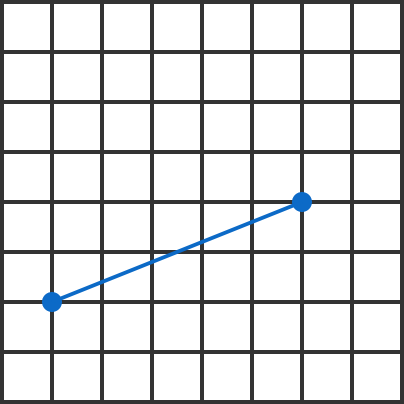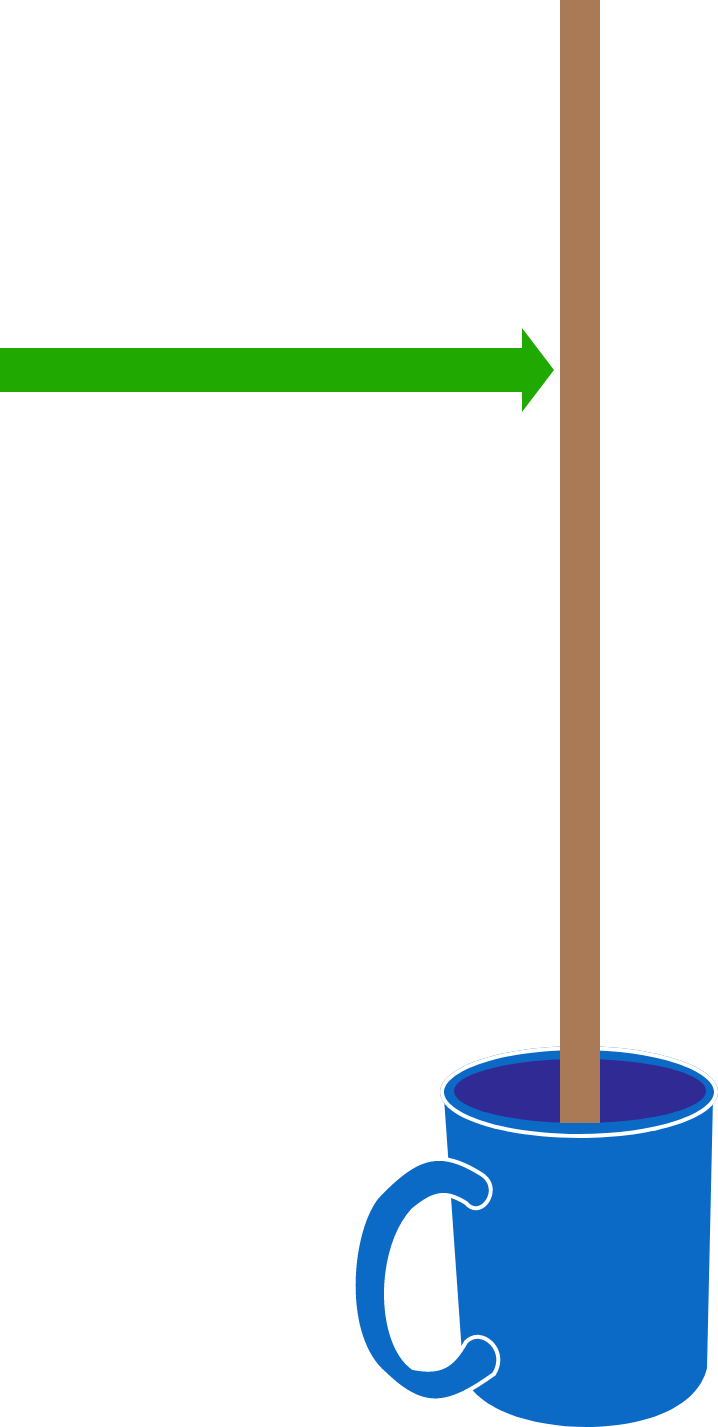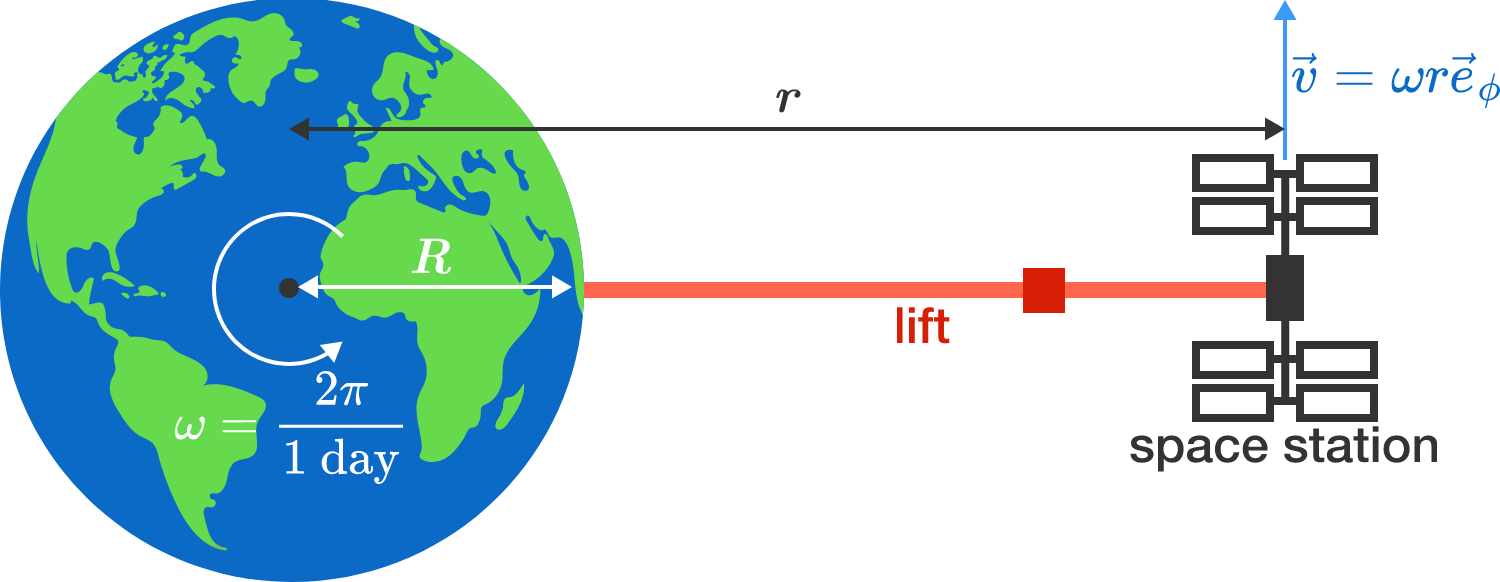# Problems of the Week

Contribute a problem

# 2017-10-02 AdvancedOn an $$n \times n$$ square grid, two vertices are chosen at random and a segment is drawn connecting them. As $$n\rightarrow \infty,$$ what is the probability that the segment does not cross any vertices of this graph besides the two chosen ones?A wooden stick of length $$\ell$$ is balanced vertically in a cup on a table. Your job is to hit the stick so that the bottom end of the stick comes out of the cup without touching the cup. If you strike it too low (close to the cup), the bottom of the stick will move forward and hit the wall of the cup. If you strike it too high, the bottom of the stick will move backward and hit the wall of the cup.

What is the distance $$h$$ from the top that you should hit the stick so that the bottom of the stick moves vertically upwards, not touching the cup?

Express the distance as $$h=a\ell,$$ and give your answer as $$a$$ to 3 decimal places.

Suppose I roll a fair, six-sided die repeatedly until I get a 6. It is well-known that the expected number of rolls is exactly 6.

Now, suppose instead that I roll a fair, six-sided die repeatedly until I get a 6, and I tell you that every time I rolled the die, an even number (2, 4, or 6) came up. What is the expected number of die rolls that I made?


Clarification: For example, I could have rolled 2-4-4-2-4-6, 2-6, 4-4-6, or just 6.

Source: Gil Kalai's blog

NASA is planning to stretch a long cable from the equator into space, so that persons and loads can be transported to the orbiting height of the space station, and that the cable would always remain above the same point on Earth. Now, a short rope would fall under its own weight to Earth, but once its length exceeds a certain limit, the rope will start to support itself due to the centrifugal force.

What minimum length must such a rope have, approximately, so that it is just carrying itself?Details and Assumptions:

• The cable is not elastic, has a uniform density, and is tightly stretched without any curvature.
• Set up the force balance for incremental rope pieces and integrate them over the length of the rope.
• The circumference of Earth is about $$2 \pi R \approx 40,000 \,\text{km}.$$
• Acceleration due to gravity g = $$10 \text{ m/s}^2.$$

Bonus question: What is the maximum tensile stress along the rope? Is there any known material that can resist these forces?

$\large f(x)=\prod _{ m=0 }^{ \infty }{\frac {\ \ \displaystyle \sum _{ n=0 }^{ m }{ { x }^{ n }\ \ } }\sum _{ n=0 }^{ \infty }{ { x }^{ n } } } }$

Given $$f(x)$$ defined as above, define $$C=\left\lfloor { 2 }^{ 120 } \times f\left(\frac { 1 }{ 2 } \right) \right\rfloor.$$ Then, how many 1's does the binary representation of $$C$$ contain? That is, what is the binary digit sum of $$C?$$


Hint: You do not need a computer to solve this problem, nor do you need to calculate $$f\left(\frac{1}{2}\right)$$ to a high accuracy. There is a more "contest-friendly" way to solve this problem.

Bonus: Can you give a formula for the digit sum of $$\left\lfloor { B }^{ 120 } \times f\left(\frac { 1 }{ B } \right) \right\rfloor$$ in base $$B?$$

×

Problem Loading...

Note Loading...

Set Loading...